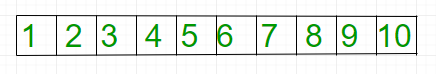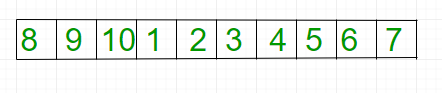# Reversal algorithm for right rotation of an array

Given an array, right rotate it by k elements.After K=3 rotationExamples:

```Input: arr[] = {1, 2, 3, 4, 5,
6, 7, 8, 9, 10}
k = 3
Output: 8 9 10 1 2 3 4 5 6 7

Input: arr[] = {121, 232, 33, 43 ,5}
k = 2
Output: 43 5 121 232 33
```

## Recommended: Please try your approach on {IDE} first, before moving on to the solution.

Note :
In the below solution, k is assumed to be smaller than or equal to n. We can easily modify the solutions to handle larger k values by doing k = k % n

Algorithm:

```rotate(arr[], d, n)
reverseArray(arr[], 0, n-1) ;
reverse(arr[], 0, d-1);
reverse(arr[], d, n-1);```

Below is the implementation of above approach:

## C++

 `// C++ program for right rotation of  ` `// an array (Reversal Algorithm) ` `#include ` ` `  `/*Function to reverse arr[]  ` `from index start to end*/` `void` `reverseArray(``int` `arr[], ``int` `start, ` `                            ``int` `end) ` `{ ` `    ``while` `(start < end) ` `    ``{ ` `        ``std::swap(arr[start], arr[end]); ` `        ``start++; ` `        ``end--; ` `    ``} ` `} ` ` `  `/* Function to right rotate arr[] ` `of size n by d */` `void` `rightRotate(``int` `arr[], ``int` `d, ``int` `n) ` `{ ` `    ``reverseArray(arr, 0, n-1); ` `    ``reverseArray(arr, 0, d-1); ` `    ``reverseArray(arr, d, n-1); ` `} ` ` `  `/* function to print an array */` `void` `printArray(``int` `arr[], ``int` `size) ` `{ ` `    ``for` `(``int` `i = 0; i < size; i++) ` `        ``std::cout << arr[i] << ``" "``; ` `} ` ` `  `// driver code ` `int` `main() ` `{ ` `    ``int` `arr[] = {1, 2, 3, 4, 5,  ` `                ``6, 7, 8, 9, 10}; ` `     `  `    ``int` `n = ``sizeof``(arr)/``sizeof``(arr); ` `    ``int` `k = 3; ` `     `  `    ``rightRotate(arr, k, n); ` `    ``printArray(arr, n); ` ` `  `    ``return` `0; ` `}  `

## Java

 `// Java program for right rotation of  ` `// an array (Reversal Algorithm) ` `import` `java.io.*; ` ` `  `class` `GFG  ` `{ ` `    ``// Function to reverse arr[]  ` `    ``// from index start to end ` `    ``static` `void` `reverseArray(``int` `arr[], ``int` `start, ` `                             ``int` `end) ` `    ``{ ` `        ``while` `(start < end) ` `        ``{ ` `           ``int` `temp = arr[start]; ` `           ``arr[start] = arr[end]; ` `           ``arr[end] = temp; ` `           ``start++; ` `           ``end--; ` `         ``} ` `    ``} ` ` `  `    ``// Function to right rotate  ` `    ``// arr[] of size n by d  ` `    ``static` `void` `rightRotate(``int` `arr[], ``int` `d, ``int` `n) ` `    ``{ ` `       ``reverseArray(arr, ``0``, n - ``1``); ` `       ``reverseArray(arr, ``0``, d - ``1``); ` `       ``reverseArray(arr, d, n - ``1``); ` `    ``} ` ` `  `    ``// Function to print an array  ` `    ``static` `void` `printArray(``int` `arr[], ``int` `size) ` `    ``{ ` `       ``for` `(``int` `i = ``0``; i < size; i++) ` `          ``System.out.print(arr[i] + ``" "``); ` `    ``} ` ` `  `    ``public` `static` `void` `main (String[] args)  ` `    ``{ ` `        ``int` `arr[] = {``1``, ``2``, ``3``, ``4``, ``5``,  ` `                     ``6``, ``7``, ``8``, ``9``, ``10``}; ` `     `  `    ``int` `n = arr.length; ` `    ``int` `k = ``3``; ` `     `  `    ``rightRotate(arr, k, n); ` `    ``printArray(arr, n); ` `         `  `    ``} ` `} ` `// This code is contributed by Gitanjali. `

## Python3

 `# Python3 program for right rotation of  ` `# an array (Reversal Algorithm) ` ` `  ` `  `# Function to reverse arr ` `# from index start to end ` `def` `reverseArray( arr, start, end): ` `     `  `    ``while` `(start < end): ` `         `  `        ``arr[start], arr[end] ``=` `arr[end], arr[start] ` `        ``start ``=` `start ``+` `1` `        ``end ``=` `end ``-` `1` `     `  ` `  `# Function to right rotate arr ` `# of size n by d  ` `def` `rightRotate( arr, d, n): ` `     `  `    ``reverseArray(arr, ``0``, n ``-` `1``); ` `    ``reverseArray(arr, ``0``, d ``-` `1``); ` `    ``reverseArray(arr, d, n ``-` `1``); ` ` `  ` `  `# function to pr an array  ` `def` `prArray( arr, size): ` `    ``for` `i ``in` `range``(``0``, size): ` `        ``print` `(arr[i], end ``=` `' '``) ` ` `  ` `  `# Driver code ` `arr ``=` `[``1``, ``2``, ``3``, ``4``, ``5``, ``6``, ``7``, ``8``, ``9``, ``10``] ` `n ``=` `len``(arr) ` `k ``=` `3` `     `  `# Function call ` `rightRotate(arr, k, n) ` `prArray(arr, n) ` ` `  ` `  `# This article is contributed  ` `# by saloni1297 `

## C#

 `// C# program for right rotation of  ` `// an array (Reversal Algorithm) ` `using` `System; ` ` `  `class` `GFG { ` `     `  `    ``// Function to reverse arr[]  ` `    ``// from index start to end ` `    ``static` `void` `reverseArray(``int` `[]arr, ``int` `start, ` `                                        ``int` `end) ` `    ``{ ` `        ``while` `(start < end) ` `        ``{ ` `            ``int` `temp = arr[start]; ` `            ``arr[start] = arr[end]; ` `            ``arr[end] = temp; ` `            ``start++; ` `            ``end--; ` `        ``} ` `    ``} ` ` `  `    ``// Function to right rotate  ` `    ``// arr[] of size n by d  ` `    ``static` `void` `rightRotate(``int` `[]arr, ``int` `d, ``int` `n) ` `    ``{ ` `        ``reverseArray(arr, 0, n - 1); ` `        ``reverseArray(arr, 0, d - 1); ` `        ``reverseArray(arr, d, n - 1); ` `    ``} ` ` `  `    ``// Function to print an array  ` `    ``static` `void` `printArray(``int` `[]arr, ``int` `size) ` `    ``{ ` `        ``for` `(``int` `i = 0; i < size; i++) ` `            ``Console.Write(arr[i] + ``" "``); ` `    ``} ` ` `  `    ``// Driver code ` `    ``public` `static` `void` `Main ()  ` `    ``{ ` `        ``int` `[]arr = {1, 2, 3, 4, 5,  ` `                    ``6, 7, 8, 9, 10}; ` `     `  `        ``int` `n = arr.Length; ` `        ``int` `k = 3; ` `     `  `        ``rightRotate(arr, k, n); ` `        ``printArray(arr, n); ` `         `  `    ``} ` `} ` ` `  `// This code is contributed by vt_m. `

## PHP

 ` `

Output:

```8 9 10 1 2 3 4 5 6 7
```

Attention reader! Don’t stop learning now. Get hold of all the important DSA concepts with the DSA Self Paced Course at a student-friendly price and become industry ready.

My Personal Notes arrow_drop_up

Improved By : chitranayal

Article Tags :
Practice Tags :

31

Please write to us at contribute@geeksforgeeks.org to report any issue with the above content.